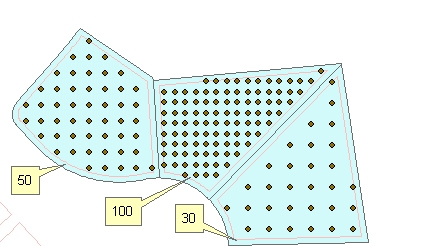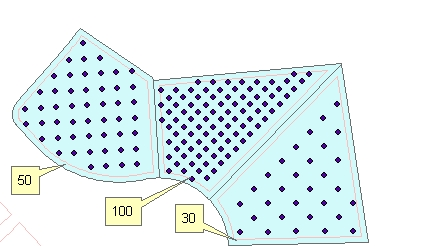Go to ET GeoWizards 12.x User Guide

Uniform Points In Polygons

Go to ToolBox Implementation

Generates uniform (regularly spaced) points located in the polygons of the input polygon dataset. The number of points per polygon can be constant or different for each polygon - based on the values in a numeric field of the input polygon feature class. The distance between the points is interpolated for each polygon. The user can specify the rotation angle for the resulting point grid.

Inputs:

• A polygon feature class
• The number of points per polygon can be input in one of the following ways
• A numeric field which values will be used to get the number of points to be generated per polygon.
• A constant number
• Optional: Minimum Distance from boundary - no point will be generated that is closer than this tolerance to the boundary of the polygon.
• Rotation angle
• Constant for all polygons - (in degrees starting from East anti-clockwise)
• From field - different rotation angle for each field (in degrees starting from East anti-clockwise)
• Along the longest axis of each polygon
• Along the longest side of each polygon

Outputs:

• New Point feature class.
• The following fields are added to the attribute table of the resulting feature class.
• [ET_ID] - the ID of the original polygon
• [ET_X] - the X coordinate of the point
• [ET_Y] - the Y coordinate of the point
• [ET_Dist] - the distance between the generated points (constant for the points in each polygon)

Illustration:

 Original polygons labeled with the values in field to be used as a source for getting the number of points to be generated and the resulting points.Rotational angle = 0, Minimum distance to boundary = 5Original polygons labeled with the values in field to be used as a source for getting the number of points to be generated and the resulting points.Rotational angle = "Along the longest Axis", Minimum distance to boundary = 5Original polygons labeled with the values in field to be used as a source for getting the number of points to be generated and the resulting points.Rotational angle = "Along the longest side", Minimum distance to boundary = 550 points per polygon.Rotational angle = "Along the longest side", Minimum distance to boundary = 0Command line syntax

ET_GPUniformPointsInPolygons<input_dataset>  <out_feature_class> {number_points_field} {number_points} {min_distance} {angle_from} {rotation_angle} {angle_field}

Parameters

Expression Explanation
<input_dataset> A Polygon feature class or feature layer
<out_feature_class> A String - the full name of the output feature class.
{number_points_field} A String representing the name of a numeric field which values will be used to get the number of points to be generated per polygon.
{number_points} A Double representing the number of points for to be created in each polygons (the same for all polygons)
{min_distance} A Double specifying the minimum distance from boundary.
{angle_from} A String defining how the rotation angle will be specified
• "Constant" - Constant for all polygons - (in degrees starting from East anti-clockwise)
• "From field" - different rotation angle for each field (in degrees starting from East anti-clockwise)
• "Longest Axis" - Along the longest axis of each polygon
• "Longest Side" - Along the longest side of each polygon
{rotation_angle} A Double - specifying the rotation angle for all polygons.
{angle_field} A String - the name of the field to be used as source for rotation angle.

Scripting syntax

ET_GPUniformPointsInPolygons(input_dataset,  out_feature_class, number_points_field, number_points, min_distance, angle_from, rotation_angle, angle_field)

See the explanations above:
<> - required parameter
{} - optional parameter